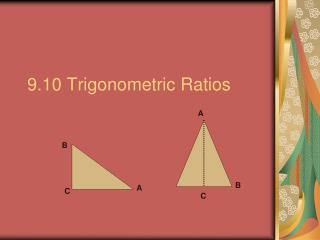Download Presentation9.10 Trigonometric Ratios

# 9.10 Trigonometric Ratios - PowerPoint PPT PresentationDownload Presentation## 9.10 Trigonometric Ratios

- - - - - - - - - - - - - - - - - - - - - - - - - - - E N D - - - - - - - - - - - - - - - - - - - - - - - - - - -
##### Presentation Transcript

1. B A C 9.10 Trigonometric Ratios A B C

2. 3 4 5 5 4 3 5 5 4 3 3 4 B A C Explore 1.Find the sine, cosine, and tangent of <A and <B in Δ ABC. 2. Compare sin <A with sin <B Compare cos <A with cos <B Compare tan <A with tan <B 3. Write a generalization that your answers to the problem above suggest. sin<A = sin<B = cos<A= cos<B = tan<A = tan<B = 5 3 4

3. Conclusion: • The sine of an angle equals the cosine of the complementary angle, or the cosine of an angle equals the sine of the complementary angle. • Also, the tangents of complementary angles are reciprocals.

4. A A H H P P Angle of Elevation • An angle of elevation is the angle formed from point P to point A. • PA (line of sight) and PH (horizontal line) is called the angle of elevation.

5. Angle of depression • If you look down from point P towards point B, the angle that PB makes with PH is called the angle of depression. P H B A

6. ATTENTION! • An angle of elevation or depression is an angle between a LINE OF SIGHT and the HORIZONTAL. • DO NOT USE THE VERTICAL!!!!!

7. For triangles other than the 30-60-90, and 45-45-90, use the table on page 424. What is the distance by land to the ship? H 28° 360m C S X Draw parallel lines from the ground. Remember alt int <‘s are congruent.

8. <CSH = 28° • Thus: tan 28 ° = 360 0.5317 = 360 0.5317X = 360 X ≈ 677 Look up tan 28 ° on the table, page 424, and plug it in to solve. x x Multiply both sides by x Divide by 0.5317 The horizontal distance is about 677 m. Use a calculator where necessary.